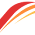### Saturated and Unsaturated Vapour Pressure

Saturated and Unsaturated Vapour Pressure What is a saturated solution? If in a water sugar solution, the amount of dissolved sugar is equal to the maximum amount that a given amount of water can dissolve at a certain temperature, then that solution is called a saturated solution. What is an unsaturated solution? If in a water sugar solution, the amount of dissolved sugar is less than that amount that a given amount of water can dissolve at a certain temperature, then that solution is called a saturated solution. What is saturated vapour? At a certain temperature in an enclosed space, if the amount of vapour present is equal to the maximum vapour capacity of that space, then that vapour is called saturated vapour. What is unsaturated vapour? At a certain temperature in an enclosed space, if the amount of vapour present is less than the maximum vapour capacity of that space, then that vapour is called unsaturated vapour. What is vapour pressure? Vapour enclosed in a container behaves

## Meaning of Concept, Law, Principle, Postulates, Hypothesis and Theory in Physics

What is Concept?

The correct realisation or understanding of a subject is to have a clear idea about that subject. Or, concept is an idea or thought or a general opinion. For example, the idea of heat is a form of energy which when applied to a body or if the body is heated, then temperature increases and on releasing heat temperature decreases.

What is Law?

When a theory is proved by different experiments and its important facts are expressed by a statement then it is called a scientific law. For example, Ohm's law, Boyle's law and sometimes on the subject matter, e.g., conservation law of energy, thermodynamic law and sometimes on both e.g., Newton's laws of motion, Galileo's laws of falling body.

What is Principle?

The natural truth which can be proved directly and by the help of that truth many natural phenomena can be established, is called a principle. For example, Doppler's principle, Heisenberg's uncertainty principle, etc.

What is Postulates?

In order to establish a mathematical model or a law, if some prior conditions are accepted, then those conditions are called postulates. Generally, a scientific theory starts with a generalised statement, it is postulate. For example, famous scientist Neils Bohr accepted two postulates to present the nuclear model. Again, scientist Einstein introduced special theory of relativity which is based on two fundamental postulates.

What is hypothesis?

In order to give explanation to their observed events, scientists consider some assumptions in conformity with previously discovered natural rules. These assumptions are called hypotheses. A hypothesis may be accepted or rejected by experiments or observations. But there are some hypotheses which, even after verification, are known as hypotheses. For example, Avogadro's hypothesis.

What is theory?

A theory is established in combination of hypothesis and rule. A hypothesis proved by experiments is called theory. For example, Einstein's theory of relativity, quantum theory, etc.

1.আপনার পোস্টটি অনেক ভালো লেগেছে আমার। আমিও আপনার মতো ভালো ব্লগার হতে চায়। প্লিজ আমার এই পোস্টটি, সুমাইয়া নামের অর্থ কি , একটু চেক করবেন। আমার জন্য দোয়া করবেন।